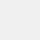# Graphing Calculator - Algeo

Version 2.33.1
+5 K
Install
Tools
Category
13 MB
Size# Graphing Calculator - Algeo

Marton Veges
Version 2.33.1
+5 K
Install
Tools
Category
13 MB
Size

## Introduction

Algeo is the most beautiful scientific graphing calculator available on the Play Store. It's fast and powerful and you'll never have to carry around a large physical TI calculator anymore. The intuitive interface shows your drawn functions as you would write them on paper rather than squeezing everything on a single line. And you don't need an internet connection unlike other graphing calculators, it works offline as well! Useful for calculus, physics or to solve those x+y equations.

This free app is packed with more features than a big TI 84 graphing calculator. Solve your homework with Algeo: draw functions, find intersections and show a table of values of the functions with an easy to use interface.

As a calculus calculator
• Symbolic Differentiation
• Calculate Integrals (definite only)
• Calculate Taylor-series
• Solve Equations
• Draw function
• Function plotting and finding roots of functions

As a scientific graphing calculator
• Trigonometric and Hyperbolic Functions
• Radians and Degree Support
• Logarithm
• Result History
• Variables
• Scientific Notation
• Combinatorial functions
• Solve linear equations (x+y)
• Do everything what you can do with a ti graphing calculator
• Number theoretic functions (modulo, greatest common divisor)

As a free graphing calculator
• Draw up to four functions
• Analyze function
• Find roots and intersections automatically
• Pinch to zoom
• Share your plots with your classmates
• Create an infinite table of values for a function

This graphing calculator is the easiest way to analyze a function and integrate and differentiate equations. Useful for math classes in high school or university. Take the Calculus quizzes with confidence knowing that Algeo helps with all mathematical functions. It makes integrating a breeze.

If you need help press the Menu button -> Help or send us an e-mail. We are happy to help with any questions!

To get the latest features faster check out our beta releases:

User Reviews - 50 Rates
4.7 from 5
5
4
3
2
1masomeh
2021/10/05
عالیهجواد
2021/08/20
خیلی عالیه من این نرم افزار رو بیشتر از ۳ ساله ک دارمش فقط میترسم آپدیتش کنم ک نکنه بعضی قسمت هاش محدود بشهsasan
2021/01/21
بسیار عالی و کاربردی